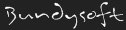L3DT documentationLarge 3D terrain generator

# Assigning land types

Determining which land type goes where in the attributes map is done by evaluating 'scores' for all the land types at every pixel in the map. The land type with the highest score at a particular heightfield pixel wins, and is assigned to the attributes map. The score for a land type is a function of several properties calculated from the heightfield and water-map, including:

• Land altitude.
• Curvature (rate of change of gradient).
• Water level (inc. water table).
• Water salinity.

Each land type has a set of coefficients for these values that determines the weighting effect in the final score for the land type (see land type definition). For example, a 'beach sand' land type might have positive coefficients for water and salinity (because you find sand near salty water), but negative coefficients for gradient and curvature (because you don't often find steep, bumpy beaches).

## The equation (or a part thereof)

The equation for used to calculate the 'score' for each land type looks like this:

score = BaseScore + gradient × cgrad + curvature × ccurv + ... etc (perlin noise, water, salinity)

Where:

• BaseScore = a baseline score for the land type (see Base score parameter in land types).
• gradient = the average (absolute) land gradient (or 1st derivative), evaluated at the point of land in question.
• cgrad is the gradient coefficient for the land type being scored.
• curvature = the average (absolute) 2nd derivative of the heightfield (gradient of gradient, also 'bumpiness'), again evaluated at the point of land in question.
• ccurv is the curvature coefficient for the land type being scored.

The additional perlin, water, and salinity terms are of a more complex nature, and will not be listed here.

l3dt/algorithms/cli/am_algo.txt · Last modified: 2017/08/31 06:33 (external edit)